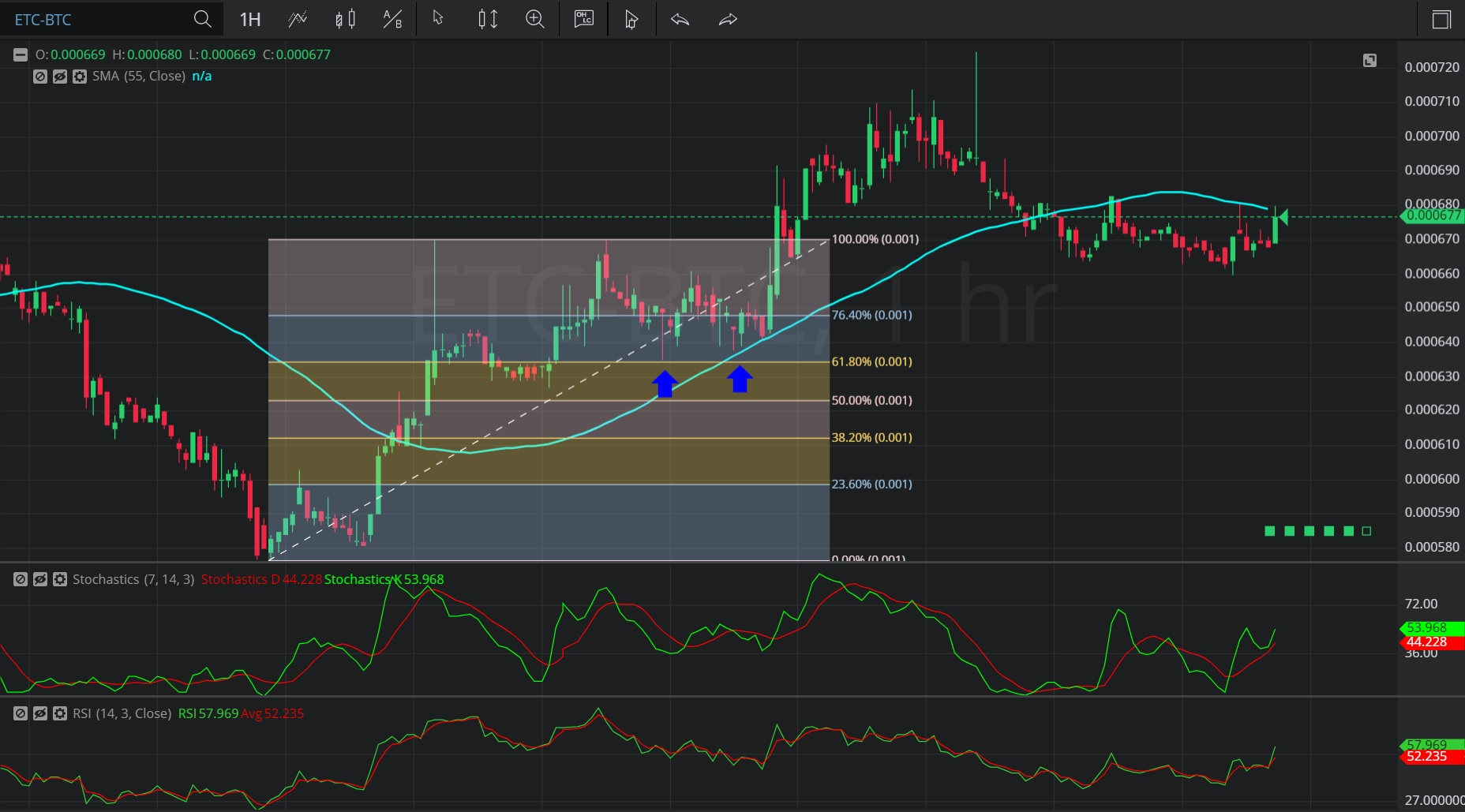Hot Topics

## 15 January 2020 - ETC/ BTC – HourlyIn this Hourly chart of Ethereum Classic/ Bitcoin (ETC/ BTC), there is an appreciating range from 0.000576 to 0.000670.

We can observe that the market then depreciated to the 0.000635 level (represented by the first blue up arrow), right around the 0.00063409 area that represents the 38.2% retracement of the appreciating range.

We can also observe that the price was then supported for several bars around the 55-bar Simple Moving Average (identified by the second blue up arrow), and that the price then appreciated to the 0.000725 level.

We can see that the price then depreciated to the 0.000660 level.

Using RSI, we can observe that the slope of RSI (14) and the slope of RSI Average (3) turned positive after the market tested the 55-bar Simple Moving Average, and that RSI (14) bullishly crossed above RSI Average (3).  The market then appreciated.

Using Slow Stochastics, we can observe that the slope of Stochastics K and the slope of Stochastics D bullishly turned positive after the market tested the 55-bar Simple Moving Average, and that Stochastics K bullishly crossed above Stochastics D.  The market then appreciated.

Stay on top of the cryptocurrency market with CryptoDaily’s trading charts!

https://charts.cryptodaily.net/